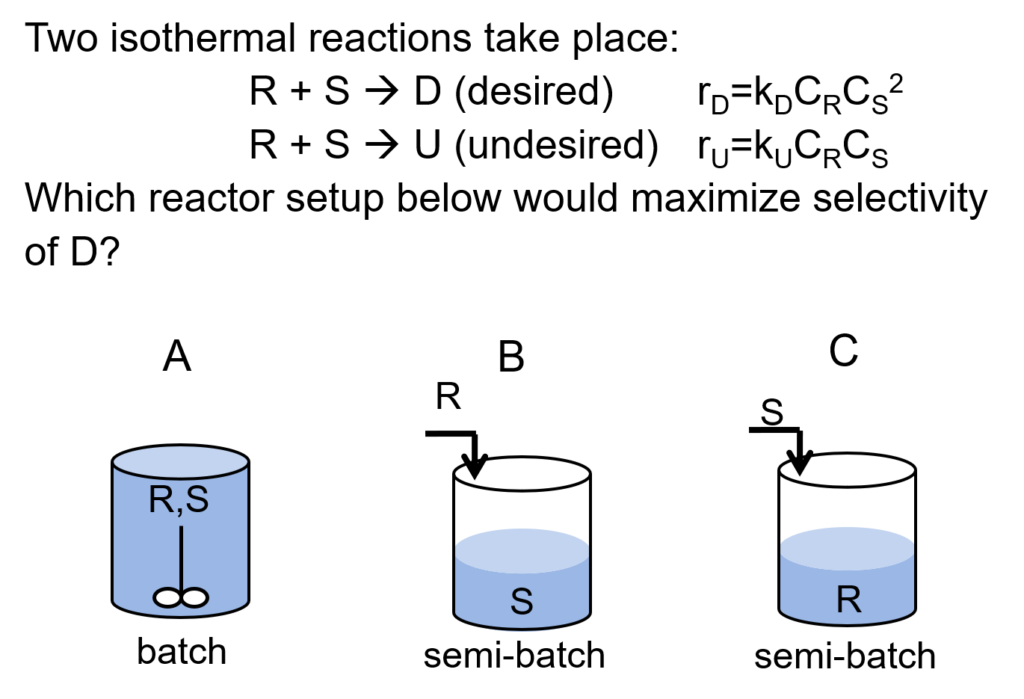LearnChemE

#### Isothermal Semibatch Reactor: ConcepTest and Example Problem

Try to answer this ConcepTest and solve this example problem before using this module. Studies show that trying to answer the questions before studying material improves learning and retention. By the end of this module, you should be able to answer these on your own. Answers will be given later in this module.##### Example Problem 1

In a semibatch reactor, we have the following mixed-phase reaction:
A(liq) + H2 → P(liq).
Determine the time to reach 80% conversion and flow of reactant as a function of time given these operating conditions:
Pressure = 3 bar, NA0 = 150 mol, -rA = kCAPH2, k = 0.0074 bar-1 min-1